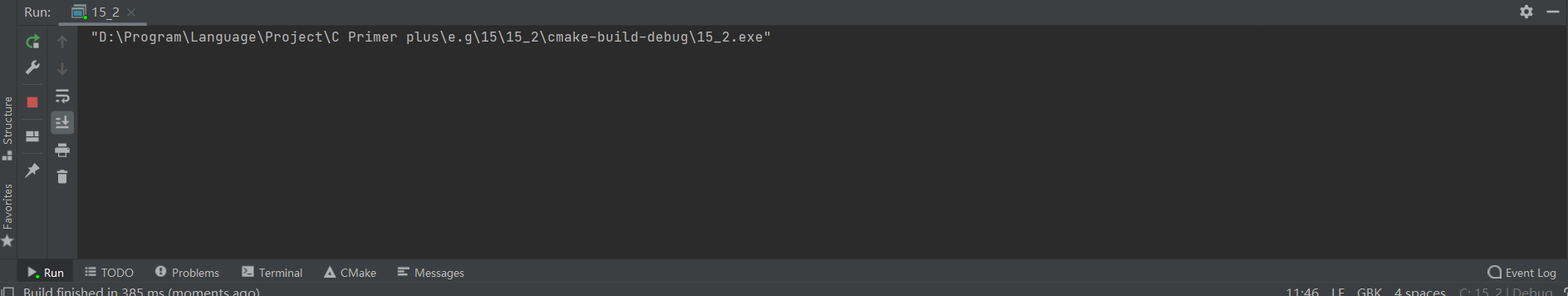2021-03-04 00:49

# CLion代码不能正常输出，可用其他编辑器就可以!!!!!

```/*invert4.c --使用位操作显示二进制*/
#include <stdio.h>
#include <limits.h>

char* itobs(int, char*);
void show_bstr(const char*);
int invert_end(int num, int bits);

int main(void)
{
char bin_str[CHAR_BIT * sizeof(int) + 1];

int number;
puts("Enter integers and see them in binary.");
puts("Non-numberic input terinates program.");

while(scanf("%d", &number) == 1)
{
itobs(number, bin_str);
printf("%d is\n", number);
show_bstr(bin_str);
putchar('\n');
number = invert_end(number, 4);
printf("Inverting the last 4 bits gives\n");
show_bstr(itobs(number, bin_str));
putchar('\n');
}
puts("Bye!");

return 0;
}

char* itobs(int n, char* ps)
{
int i;
const static int size = CHAR_BIT * sizeof(int);

for(i = size - 1; i >= 0; i--, n >>= 1)
ps[i] = (01 & n) + '0';
ps[size] = '\0';

return ps;
}

/*你4位为一组， 显示二进制字符串*/
void show_bstr(const char * str)
{
int i = 0;

while(str[i])
{
putchar(str[i]);
if(++i % 4 == 0 && str[i])
putchar(' ');
}
}

int invert_end(int num, int bits)
{
int mask = 0;
int bitval = 1;

while (bits-- > 0)
{
mask |= bitval;
bitval <<= 1;
}

return num ^ mask;
}```

附上我的输出结果• 点赞
• 写回答
• 关注问题
• 收藏
• 复制链接分享
• 邀请回答